0731-82272030# JavaScript专题之函数记忆

2015年08月16日 湖南景煌网络科技有限公司 www.0731jianzhan.com

// (来自《JavaScript指南》) function memoize(f) { var cache = {}; return function(){ var key = arguments.length + Array.prototype.join.call(arguments, ","); if (key in cache) { return cache[key]        } else return cache[key] = f.apply(this, arguments)    }}

var add = function(a, b, c) { return a + b + c} var memoizedAdd = memorize(add) console.time("use memorize") for(var i = 0; i < 100000; i++) {    memoizedAdd(1, 2, 3)} console.timeEnd("use memorize") console.time("not use memorize") for(var i = 0; i < 100000; i++) {    add(1, 2, 3)} console.timeEnd("not use memorize")

var propValue = function(obj){ return obj.value} var memoizedAdd = memorize(propValue) console.log(memoizedAdd({value: 1})) // 1 console.log(memoizedAdd({value: 2})) // 1

// (来自 underscore 的实现) var memorize = function(func, hasher) { var memoize = function(key) { var cache = memoize.cache; var address = "" + (hasher ? hasher.apply(this, arguments) : key); if (!cache[address]) {            cache[address] = func.apply(this, arguments);        } return cache[address];    };    memoize.cache = {}; return memoize;};

var count = 0; var fibonacci = function(n){    count++; return n < 2? n : fibonacci(n-1) + fibonacci(n-2);}; for (var i = 0; i <= 10; i++){    fibonacci(i)} console.log(count) // 453

var count = 0; var fibonacci = function(n) {    count++; return n < 2 ? n : fibonacci(n - 1) + fibonacci(n - 2);};fibonacci = memorize(fibonacci) for (var i = 0; i <= 10; i++) {    fibonacci(i)} console.log(count) // 12

1. 我们的承诺
2. 我们的实力
3. 我们的未来
•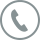7*24小时售后支持

全国7x24小时客服热线

•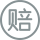50倍故障时长赔偿

50倍赔付终端服务时间

•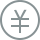7天无理由退款

部分产品可享受15天无理由退款

•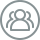优秀的团队服务

全国多家分公司便捷服务

•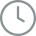10年历史
•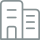多家分公司
•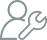高级设计师、工程师
•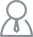过万客户
•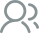同行

持续陪伴企业成长，共创，共赢

•赋能

帮助企业建设全网营销生态链

•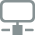连接

让企业与用户快速连接一切

•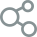未来

为企业与用户搭建共享开发平台全国服务热线

0731-82272030